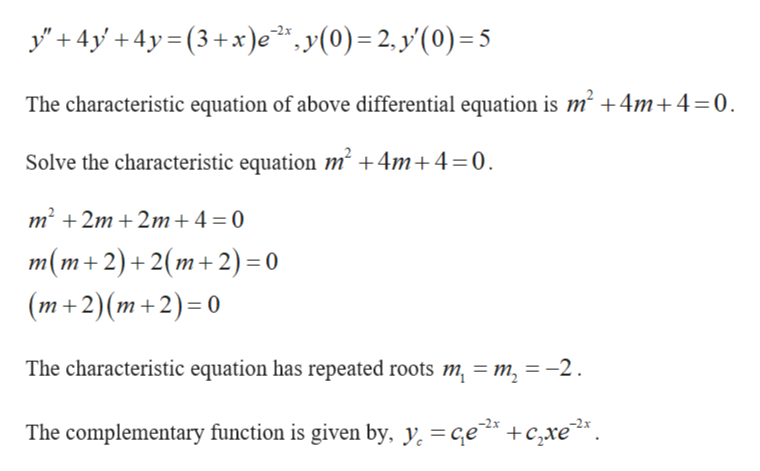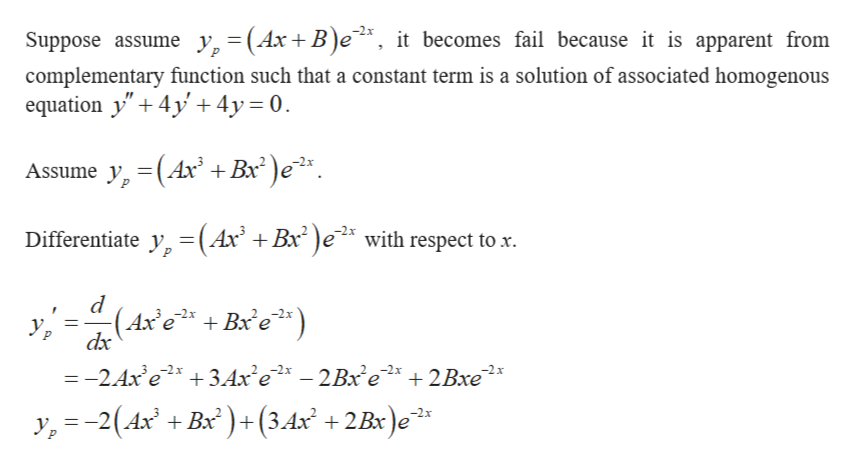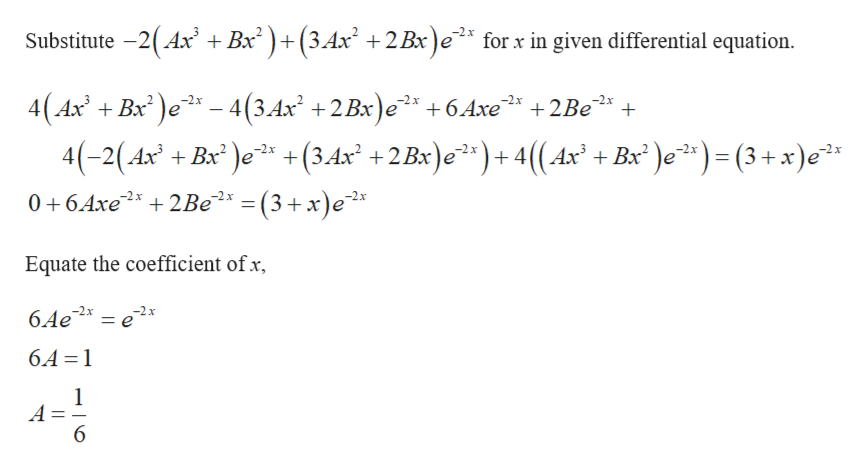Solve the initial value problem using the method of undetermined coefficients. y"+4y'+4y=(3+x)e-2x, y(0)=2, y'(0)=5

Question

Solve the initial value problem using the method of undetermined coefficients.

y"+4y'+4y=(3+x)e-2x, y(0)=2, y'(0)=5

Step 1

The given intial value problem is given by,help_outlineImage Transcriptionclosey4+4y3+x)e, y(0) = 2. y'(0) 5 The characteristic equation of above differential equation is m2 +4m+ 4=0 Solve the characteristic equation m2 +4m+4 = 0 m2 2m 2m+ 4 = 0 m(m+2)+2(m+2) = 0 (m+2)(m+2)= 0 The characteristic equation has repeated roots m m, = -2 The complementary function is given by, y. = ce' +С хе. fullscreen
Step 2

Find particular solution.help_outlineImage TranscriptioncloseSuppose assume y,=(Ax+ B)e, it becomes fail because it is apparent from complementary function such that a constant term is a solution of associated homogenous equation y" 4y 4y 0 Assume y, (Ax +B )e Differentiate y (Ax' +Bx2)e2* with respect to x (Axe +Bxe dx Ур —-2 Ах е? + ЗАх'е — 2Вх е* + 2Вхе y,-2(4x + Bx)(3.4x? +2Bx)e*: fullscreen
Step 3

Use substitution to find p...help_outlineImage TranscriptioncloseSubstitute -2(Ax Bx+(34x +2 Bx)e2 2x for x in given differential equation 4(Ax Bx)e-4(3.4x +2Bx)e +6 Axe 4(-2(4x -2x -2x +2Be )e*) = (3 + x)c* x* )e + (3,4x +2 Bx)e2)+4((4x + Bx = (3+ x)e -2x 0 6Axe22Be -2 x Equate the coefficient of x -2x 6.Ae 6.A 1 1 A = 6 fullscreen

Want to see the full answer?

See Solution

Want to see this answer and more?

Our solutions are written by experts, many with advanced degrees, and available 24/7

See Solution
Tagged in

Math